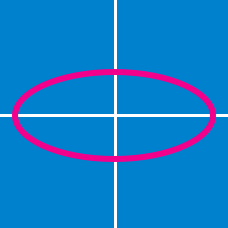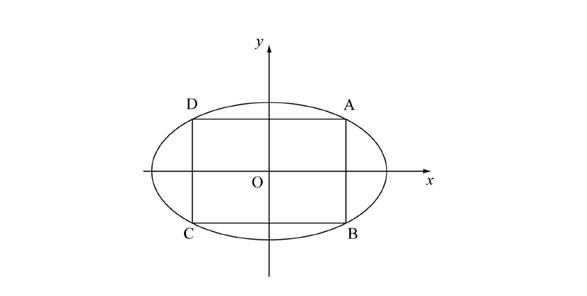Geometry

# Conics - Ellipse - General

Let $(m,n)$ be the point at which a line is tangent to the ellipse $\displaystyle{\frac{x^2}{16}+\frac{y^2}{100}=1}.$ If this line has $x$-intercept $A=(a,0)$ and $y$-intercept $B=(0,b),$ where $a$ and $b$ are positive, what is the minimum value of $ab?$

The ellipse $\displaystyle{\frac{x^2}{8}+\frac{y^2}{17}=1}$ and the line $y=2x - k$ intersect at exactly two distinct points. What is the range of the constant $k?$

Find the equation of the tangent line to the ellipse $1x^2+y^2=53$ at the point $(2,7)$ on the ellipse.In the above diagram, a rectangle $ABCD$ is inscribed in the ellipse $\displaystyle{\frac{x^2}{36}+\frac{y^2}{100}=1}.$ What is the maximum area of rectangle $ABCD?$

Note: The above diagram is not drawn to scale.

Find the maximum area of the rectangle which is inscribed in the ellipse $\frac{x^{2}}{5^{2}}+\frac{y^{2}}{2^{2}} = 1.$

×